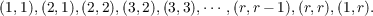#### Vol. 27, No. 1, 1968

 Download this articleFor screen For printingRecent Issues Vol. 323: 1  2 Vol. 322: 1  2 Vol. 321: 1  2 Vol. 320: 1  2 Vol. 319: 1  2 Vol. 318: 1  2 Vol. 317: 1  2 Vol. 316: 1  2Online Archive Volume: Issue:The Journal Subscriptions Editorial Board Officers Contacts Submission Guidelines Submission Form Policies for Authors ISSN: 1945-5844 (e-only) ISSN: 0030-8730 (print) Special Issues Author Index To Appear Other MSP Journals
On QF-1 algebras

### Denis Ragan Floyd

Vol. 27 (1968), No. 1, 81–94
##### Abstract

Let A be a finite-dimensional associative algebra with identity over a field k, M an A-module which is finite-dimentional as a vector space over k, and E = Homk(M,M) the algebra of linear transformations on M. For a A. Let aL denote the linear transformation of M given by aL(x) = ax, for x M. Define the following subalgebras of E:

 AL = {aL : a ∈ A} C = {f ∈ E : f(ax) = af(x) for each a ∈ A,x ∈ M} D = {f ∈ E : f(g(x)) = g(f(x)) for each g ∈ C,x ∈ M}.

Clearly, AL D. Require M to be faithful. Then A is isomorphic to, and will be identified with, AL. If A = D, it is said that the pair (A,M) has the double centralizer property.

A is called a QF-1 algebra if (A,M) has the double centralizer property for each faithful A-module M.

The following results in the theory of QF-1 algebras are obtained:

1. Let A be a commutative algebra over an arbitrary field. Then A is QF-1 if and only if A is Frobenius.

2. Let A be an algebra such that the simple left A-modules are one-dimensional. Suppose there exist distinct simple two-sided ideals A1 and A2 contained in the radical of A, and primitive idempotents e and f, such that eAkf0, for k = 1,2. Then A is not QF-1.

3. Let A be an algebra with the properties that the simple left A-modules are one-dimensional, and the two-sided ideal lattice of A is distributive. Then if A satisfies any one of the following conditions, it is not QF-1.

(a) There exist, for r 2, 2r distinct simple two-sided ideals Auv contained in the radical, and primitive idempotents eiu and ejv for 1 u, v r, satisfying eiuAuvEjv0, where the index pair (u,v) ranges over the set(b) There exist, for r 1, 2r + 2 distinct simple two-sided ideals Auv and Avρ, for (u,v) = (1,1),(1,2),,(r 1,r 1),(r 1,r), and (ρ,v) = (1,1),(2,1),(3,r), and (4,r), and primitive idempotents eiu, ejv, and ekρ satisfying eiuAuvej0 and ekρAvρejv0, where (u,v) and (ρ,v) range over the index pairs indicated above.

Primary: 16.50
##### Milestones